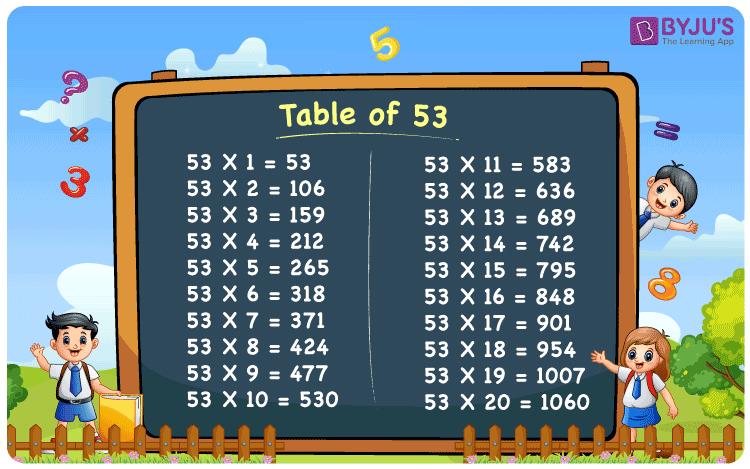# Table of 53

The Table of 53, also known as the 53 times table, is the multiplication table that results in the product of the number 53 with consecutive natural numbers. Learning the multiplication table of 53 will assist students in saving a significant amount of time during the examination. Also, 53 times table is provided in the PDF, so that students can download the multiplication table and learn offline as well.

## Table of 53 Chart

The table of 53 chart is provided here for students for faster calculation.## What is the 53 Times Table?

The 53 times table is the repeated addition of number 53 for “n” number of times, where “n” represents the natural number. Go through the below 53 times table provided here up to the first 10 times, to understand it quickly.

 53 × 1 = 53 53 53 × 2 = 106 53 + 53 = 106 53 × 3 = 159 53 + 53 + 53 = 159 53 × 4 = 212 53 + 53 + 53 + 53 = 212 53 × 5 = 265 53 + 53 + 53 + 53 + 53 = 265 53 × 6 = 318 53 + 53 + 53 + 53 + 53 + 53 = 318 53 × 7 = 371 53 + 53 + 53 + 53 + 53 + 53 + 53 = 371 53 × 8 = 424 53 + 53 + 53 + 53 + 53 + 53 + 53 + 53 = 424 53 × 9 = 477 53 + 53 + 53 + 53 + 53 + 53 + 53 + 53 + 53 = 477 53 × 10 = 530 53 + 53 + 53 + 53 + 53 + 53 + 53 + 53 + 53 + 53 = 530

## Multiplication Table of 53

Below is the multiplication table of 53 up to 20 times. Go through the below table and solve the problems quickly.

 53 × 1 = 53 53 × 2 = 106 53 × 3 = 159 53 × 4 = 212 53 × 5 = 265 53 × 6 = 318 53 × 7 = 371 53 × 8 = 424 53 × 9 = 477 53 × 10 = 530 53 × 11 = 583 53 × 12 = 636 53 × 13 = 689 53 × 14 = 742 53 × 15 = 795 53 × 16 = 848 53 × 17 = 901 53 × 18 = 954 53 × 19 = 1007 53 × 20 = 1060

## Solved Examples on the Table of 53

Example 1:

Find the value of 53 times 15 plus 100.

Solution:

53 times 15 = 53 × 15 = 795

Adding 100 to 795, we get 895.

I.e., 795 + 100 = 895.

Therefore, the value of 53 times 15 plus 100 is 895.

Example 2:

Ramya purchased a book that costs Rs. 53. Find the total cost of the book, if she purchased 10 books.

Solution:

The cost of 1 book = Rs. 53.

So, the cost of 10 books  = 10 × 53 = 530

Therefore, the cost of 10 books is Rs. 530.

## Frequently Asked Questions on the Table of 53

### What is the table of 53?

The table of 53 is the multiplication of the number 53 with the natural numbers. The table of 53 is also known as the 53 times table.

### Write the table of 53 up to 10 times.

The table of 53 up to 10 times are as follows: 53 × 1 = 53, 53 × 2 = 106, 53 × 3 = 159, 53 × 4 = 212, 53 × 5= 265, 53 × 6 = 318, 53 × 7 = 371, 53 × 8 = 424, 53 × 9 = 477, 53 × 10 = 530

### What is 53 times 9?

53 times 9 is 477. I.e., 53 × 9 = 477.

### What is 53 times 14?

53 times 14 is 742. I.e., 53 × 14 = 742.